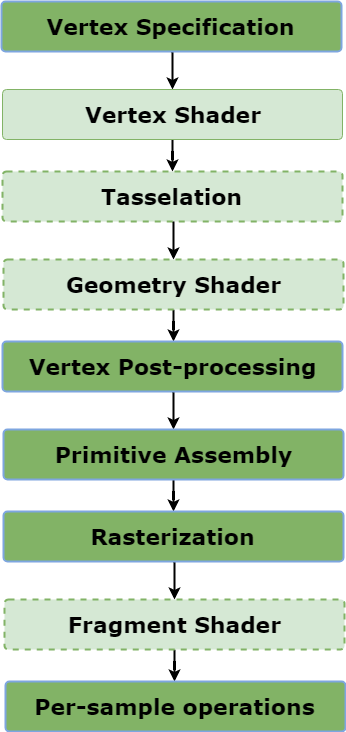GeeksforGeeks App
Open AppBrowser
Continue

# OpenGL Rendering Pipeline | An Overview

Rendering Pipeline is the sequence of steps that OpenGL takes when rendering objects. Vertex attribute and other data go through a sequence of steps to generate the final image on the screen. There are usually 9-steps in this pipeline most of which are optional and many are programmable.

Sequence of steps taken by openGL to generate an image :1. Vertex Specification : In Vertex Specification, list an ordered list of vertices that define the boundaries of the primitive. Along with this, one can define other vertex attributes like color, texture coordinates etc. Later this data is sent down and manipulated by the pipeline.
2. Vertex Shader : The vertex specification defined above now pass through Vertex Shader. Vertex Shader is a program written in GLSL that manipulate the vertex data. The ultimate goal of vertex shader is to calculate final vertex position of each vertex. Vertex shaders are executed once for every vertex(in case of a triangle it will execute 3-times) that the GPU processes. So if the scene consists of one million vertices, the vertex shader will execute one million times once for each vertex. The main job of a vertex shader is to calculate the final positions of the vertices in the scene.
3. Tessellation : This is a optional stage. In this stage primitives are tessellated i.e. divided into smoother mesh of triangles.
4. Geometry Shader : This shader stage is also optional. The work of Geometry Shader is to take an input primitive and generate zero or more output primitive. If a triangle strip is sent as a single primitive, geometry shader will visualize a series of triangles. Geometry Shader is able to remove primitives or tessellate them by outputting many primitives for a single input. Geometry shaders can also convert primitives to different types. For example, point primitive can become triangles.
5. Vertex Post Processing : This is a fixed function stage i.e. user has a very limited to no control over these stages. The most important part of this stage is Clipping. Clipping discards the area of primitives that lie outside the viewing volume.
6. Primitive Assembly : This stage collects the vertex data into a ordered sequence of simple primitives(lines, points or triangles).
7. Rasterization : This is an important step in this pipeline. The output of rasterization is a fragments.
8. Fragment Shader : Although not necessary a required stage but 96% of the time it is used. This user-written program in GLSL calculates the color of each fragment that user sees on the screen. The fragment shader runs for each fragment in the geometry. The job of the fragment shader is to determine the final color for each fragment.
9. Per-sample Operations : There are few tests that are performed based on user has activated them or not. Some of these tests for example are Pixel ownership test, Scissor Test, Stencil Test, Depth Test.

Check out rendering a simple triangle using openGL here.

My Personal Notes arrow_drop_up